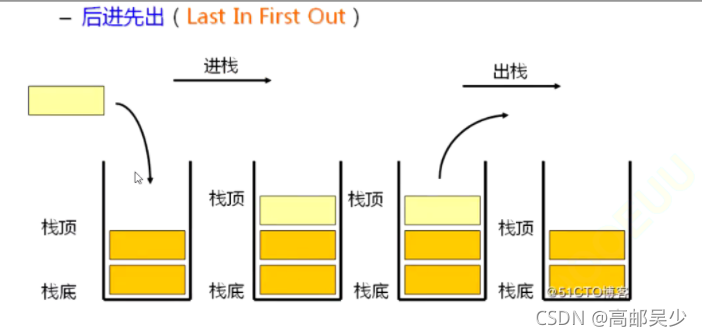﻿ C语言编程数据结构栈与队列的全面讲解示例教程_C 语言_脚本之家
C 语言# C语言编程数据结构栈与队列的全面讲解示例教程

## 一、栈的表示和实现

### 1栈的概念和结构（图片来自比特就业课）

```typedef int STDataType;//方便将来如果需要其他类型的数据，可以直接修改int的类型
typedef struct Stack
{
STDataType*a;//栈底指针
int top;//栈顶标号
int capacity;//容量
}ST;
```

### 2栈的初始化

```#include<assert.h>
#include<stdlib.h>//malloc函数头文件
void StackInit(ST*ps)//栈初始化
{
assert(ps);//防止传过来的指针是空指针
ps->a = (STDataType)malloc(sizeof(STDataType) * 4);//malloc开辟一块空间出来，由STDataType类型进行管理
//malloc，及后文出现的realloc、free函数详情见笔者动态内存管理文章
if (ps->a == NULL)
{
printf("realloc fail\n");
exit(-1);//终止程序
}
ps->capacity=4;
//这里以开辟4个数据容量大小的栈为例，你也可以写其他数字
//如果压栈的时候内存不够，可以在后面提到的压栈函数里面进行扩容
ps->top = 0;//刚开始栈里没有值时，用top=0标记，后续每放入一个top++
//关于top详细用法请往下看1.3压栈部分
}
```

### 3压栈(栈顶插入一个数据)1进入栈之后，1就是栈顶元素了，那如果我想继续入栈，就是要在top的位置放入一个数据让新放入的数据成为新的栈顶元素，然后以此类推，每次入一个元素，top++，top不是表示栈顶元素位置，而是栈顶元素下一个位置。```#include<assert.h>//assert函数头文件
#include<stdlib.h>//realloc函数头文件
void StackPush(ST*ps, STDataType x)//栈顶插入数据（入栈）
{
assert(ps);
if (ps->top == ps->capacity)
{
STDataType*tmp = realloc(ps->a, ps->capacity * 2 * sizeof(STDataType));
//realloc是在原先开辟的空间上继续往后开辟一块空间，详细见笔者动态内存文章
//扩容一般扩2倍
if (tmp == NULL)//扩容失败（比如内存已经不够你再开辟空间了）会返回空指针
{
printf("realloc fail\n");
exit(-1);//终止程序
}
else
{
ps->a = tmp;
ps->capacity *= 2;
}
}
ps->a[ps->top] = x;//a是一个指针，a[x]==*(a+x)
ps->top++;
}
```

### 4出栈(栈顶删除一个数据)```void StackPop(ST*ps)//栈顶删除数据（出栈）
{
assert(ps);
assert(ps->top > 0);//栈空了，调用Pop，直接中止程序报错
ps->top--;
}
```### 5取栈顶元素

```STDataType StackTop(ST*ps)//取栈顶数据
{
assert(ps);
assert(ps->top > 0);//栈空了，调StackTop，直接中止程序报错
return ps->a[ps->top - 1];//top是栈顶元素下一个位置,top-1是栈顶元素
//a[m]==*(a+m)
}
```

a[ps->top - 1]你也可以写成*(a+(ps->top - 1)),这个写法读者可参加笔者以前的指针文章，这里不再赘述。

### 6取栈顶元素

```int StackSize(ST*ps)//栈的数据个数
{
assert(ps);
return ps->top;
}
```### 7判断栈是否为空

```int StackEmpty(ST*ps)//判断栈是不是空
{
assert(ps);
return ps->top == 0;
}
```

## 二、队列的表示和实现

### 1队列的概念及结构（图片来自比特就业课）

### 2队列的实现```typedef int QDataType;
typedef struct QueueNode
{
struct QueueNode*next;
QDataType data;
}QNode;//这里和单链表定义一样，有需要的可以看一下笔者之前的单链表文章
typedef struct Queue//定义一个结构体存储头节点地址和尾节点地址，方便后面头删和尾插
{
QNode*tail;
}Queue;
```

### 3队列初始化

```void QueueInit(Queue*pq)队列初始化,pq是一个结构体指针
{
assert(pq);//判断pq是否是空指针
pq->tail = NULL;
}
```

### 4入队（队尾插入一个数据）

```void QueuePush(Queue*pq, QDataType x)//入队（队尾入）
{
assert(pq);
QNode*newnode = (QNode*)malloc(sizeof(QNode));//开辟出一块空间给newnode
if (newnode == NULL)//有可能剩余内存不够开辟空间，malloc开辟失败会返回空指针
{
printf("开辟空间失败\n");
exit(-1);//退出程序
}
newnode->data = x;
newnode->next = NULL;
if (pq->tail == NULL)//原先队列里没有任何数据，头和尾指针都指向NULL
{
}
else
{
pq->tail->next = newnode;
pq->tail = newnode;
}
}
```

```    if (pq->tail == NULL)
{
}
else
{
pq->tail->next = newnode;
pq->tail = newnode;
}
```### 5出队（队头删除一个数据）

```void QueuePop(Queue*pq)//出队（队头出）
{
assert(pq);
//原队列只有1个数据
{
}
//原队列有多个数据
else
{
}
}
```

1.原队列只有1个数据2.原队列有多个数据### 6取队头数据

```QDataType QueueFront(Queue*pq)//取队头数据
{
assert(pq);
}
```

### 7取队尾数据

```QDataType QueueBack(Queue*pq)//取队尾数据
{
assert(pq);
return pq->tail->data;
}
```

### 8计算队列中数据个数

```int QueueSize(Queue*pq)//队内数据个数
{
assert(pq);
int size = 0;
while (cur)//cur!=NULL进行循环,NULL是遍历完tail之后出现的
{
size++;
cur = cur->next;
}
return size;
}
```

### 9判断队列是否为空

```bool QueueEmpty(Queue*pq)
{
assert(pq);
}
```

### 10销毁队列

```void QueueDestory(Queue*pq)//队列销毁
{
assert(pq);
while (cur)//cur!=NULL进行循环,NULL是遍历完tail之后出现的
{
QNode*next = cur->next;
free(cur);//free是释放指向的空间，指针还是在的
cur = next;
}